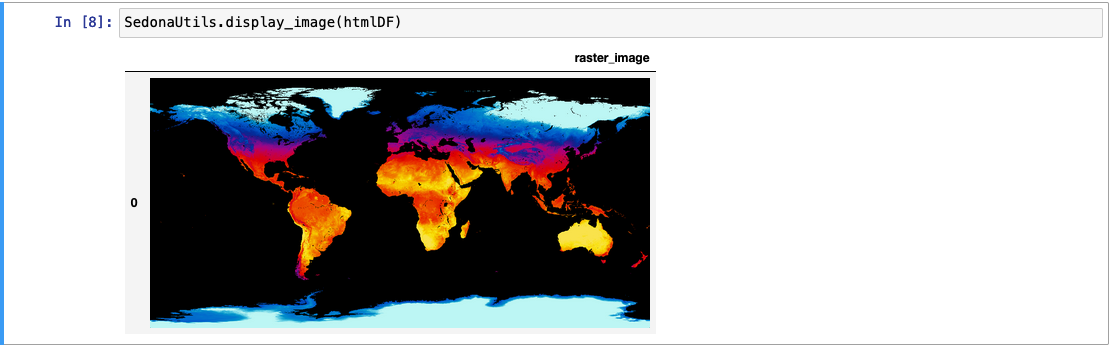# Raster visualization

Sedona offers some APIs to aid in easy visualization of a raster object.

## Image-based visualization¶

Sedona offers APIs to visualize a raster in an image form. This API only works for rasters with byte data, and bands <= 4 (Grayscale - RGBA). You can check the data type of an existing raster by using RS_BandPixelType or create your own raster by passing 'B' while using RS_MakeEmptyRaster.

### RS_AsBase64¶

Introduction: Returns a base64 encoded string of the given raster. This function internally takes the first 4 bands as RGBA, and converts them to the PNG format, finally produces a base64 string. To visualize other bands, please use it together with `RS_Band`. You can take the resulting base64 string in an online viewer to check how the image looks like.

Format: `RS_AsBase64(raster: Raster)`

Since: `v1.5.0`

Spark SQL Example:

``````SELECT RS_AsBase64(raster) from rasters
``````

Output:

``````iVBORw0KGgoAAAA...
``````

### RS_AsImage¶

Introduction: Returns a HTML that when rendered using an HTML viewer or via a Jupyter Notebook, displays the raster as a square image of side length `imageWidth`. Optionally, an imageWidth parameter can be passed to RS_AsImage in order to increase the size of the rendered image (default: 200).

Format: `RS_AsImage(raster: Raster, imageWidth: Integer = 200)`

Since: `v1.5.0`

Spark SQL Example:

``````SELECT RS_AsImage(raster, 500) from rasters
SELECT RS_AsImage(raster) from rasters
``````

Output:

``````"<img src=\"data:image/png;base64,iVBORw0KGgoAAAANSUhEUgAAAAUAAAAECAAAAABjWKqcAAAAIElEQVR42mPgPfGfkYUhhfcBNw+DT1KihS6DqLKztjcATWMFp9rkkJgAAAAASUVORK5CYII=\" width=\"200\" />";
``````

Tip

RS_AsImage can be paired with SedonaUtils.display_image(df) wrapper inside a Jupyter notebook to directly print the raster as an image in the output, where the 'df' parameter is the dataframe containing the HTML data provided by RS_AsImage

Example:

``````df = sedona.read.format('binaryFile').load(DATA_DIR + 'raster.tiff').selectExpr(\"RS_FromGeoTiff(content) as raster\")
htmlDF = df.selectExpr(\"RS_AsImage(raster, 500) as raster_image\")
SedonaUtils.display_image(htmlDF)
``````## Text-based visualization¶

### RS_AsMatrix¶

Introduction: Returns a string, that when printed, outputs the raster band as a pretty printed 2D matrix. All the values of the raster are cast to double for the string. RS_AsMatrix allows specifying the number of digits to be considered after the decimal point. RS_AsMatrix expects a raster, and optionally a band (default: 1) and postDecimalPrecision (default: 6). The band parameter is 1-indexed.

Note

If the provided band is not present in the raster, RS_AsMatrix throws an IllegalArgumentException

Note

If the provided raster has integral values, postDecimalPrecision (if any) is simply ignored and integers are printed in the resultant string

Note

If you are using `show()` to display the output, it will show special characters as escape sequences. To get the expected behavior use the following code:

``````println(df.selectExpr("RS_AsMatrix(rast)").sample(0.5).collect().mkString("\n"))
``````
``````System.out.println(String.join("\n", df.selectExpr("RS_AsMatrix(rast)").sample(0.5).collect()))
``````
``````print("\n".join(df.selectExpr("RS_AsMatrix(rast)").sample(0.5).collect()))
``````

The `sample()` function is only there to reduce the data sent to `collect()`, you may also use `filter()` if that's appropriate.

Format:

``````RS_AsMatrix(raster: Raster, band: Integer = 1, postDecimalPrecision: Integer = 6)
``````

Since: `1.5.0`

Spark SQL Example:

``````val inputDf = Seq(Seq(1, 3.333333, 4, 0.0001, 2.2222, 9, 10, 11.11111111, 3, 4, 5, 6)).toDF("band")
print(inputDf.selectExpr("RS_AsMatrix(RS_AddBandFromArray(RS_MakeEmptyRaster(1, 'd', 4, 3, 0, 0, 1, -1, 0, 0, 0), band, 1, 0))").sample(0.5).collect()(0))
``````

Output:

``````| 1.00000   3.33333   4.00000   0.00010|
| 2.22220   9.00000  10.00000  11.11111|
| 3.00000   4.00000   5.00000   6.00000|
``````

Spark SQL Example:

``````val inputDf = Seq(Seq(1, 3, 4, 0, 2, 9, 10, 11, 3, 4, 5, 6)).toDF("band")
print(inputDf.selectExpr("RS_AsMatrix(RS_AddBandFromArray(RS_MakeEmptyRaster(1, 'i', 4, 3, 0, 0, 1, -1, 0, 0, 0), band, 1, 0))").sample(0.5).collect()(0))
``````

Output:

``````| 1   3   4   0|
| 2   9  10  11|
| 3   4   5   6|
``````

Last update: November 11, 2023 03:16:06